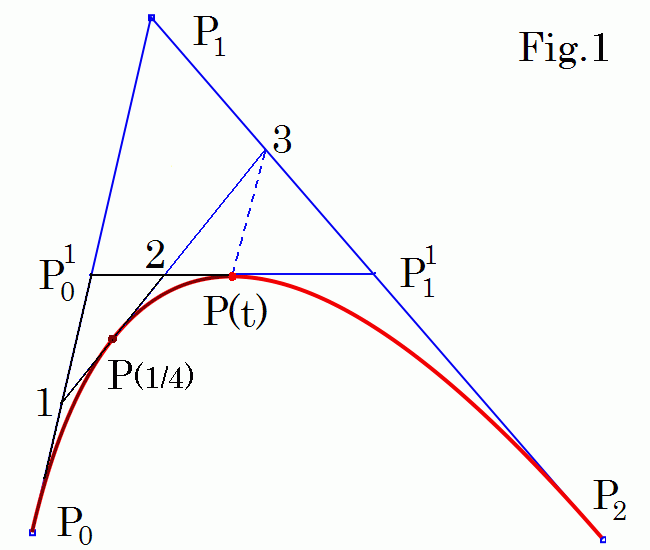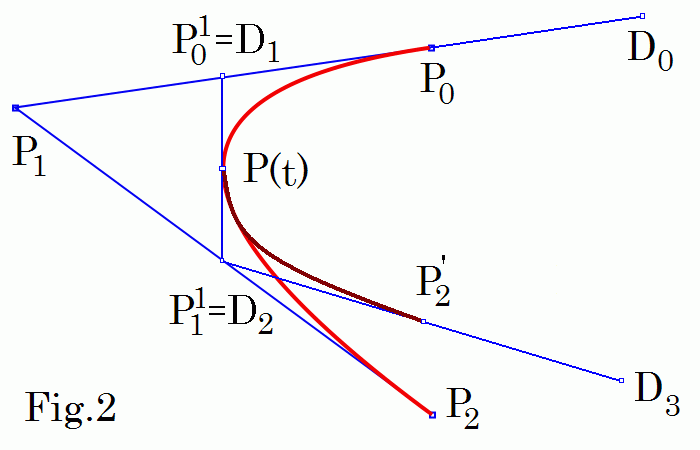# Building quadratic B-spline

The problems with a single Bezier spline range from the need of a high degree curve to accurately fit a complex shape, which is inefficient to process. To overcome the problems, a piecewise curve is used. Therefore the order of the curve is not dependent on the number of control points.

Building of piecewise B-splines is motivated below. You can skip this and go directly to the explanation of B-splines calculations.

## Quadratic Bezier spline subdivisionAny spline point P(t) subdivides Bezier curve in two smaller quadratic splines (with control points P0 , P01, P(t) and P(t), P11, P2 for quadratic splines to the left). The new curves match the original in position, although they differ in parameterization. E.g. for t = 1/2 you see to the left that triangles (1 P01 2) and (3 P(t) 2) are equal and the point P(1/4) of the original spline coincides with the point P(t') of the first small spline for t' = 1/2.

## Building complex B-spline curvesThe first derivatives of these two small splines at P(t) are determined by the P11 P(t) and P(t) P01 control segments. Therefore if we drag only P0 or P2 points we get continuous at P(t) curve. Note that both small splines depend only on its own control points. In a similar way we get piecewise B-spline with continuous (n - 2)-order derivatives from n-order Bezier spline by subdivision and end control points movement. We can repeat subdivisions to get very complex curve.

## De Boor points and Cox - be Boor algorithm

As since only 4 control points of the two small quadratic Bezier splines are independent therefore it is convenient to use 4 de Boor control points D0,1,...,3 (see Fig.2). Then one can evaluate points of k-order B-spline by the Cox - be Boor algorithm similar to the de Casteljau iterations
Pij(t) = (1 - τij)Pi-1j-1 + τij Pij-1,     Pi0 = Di ,     τij = (t - ti )/(ti+k-j - ti ),     0 < j < k,
where (t0 , ... , tn+k ) are knots and tk-1 ≤ t ≤ tn+1 .

For the spline in Fig.2 we have n = 3, k = 3, we take the uniform knot vector (-2, -1, 0, 1, 2, 3, 4). For the first spline segment D0 = P0 + (P0 - P1 ),   0 ≤ t ≤ 1 and you can check that
τ11 = (t - t1 )/(t3 - t1 ) = (t + 1)/2,
P11 = (1 - τ11)D0 + τ11D1 = (1 - t)B0 + t B1 ,
τ21 = (t - t2 )/(t4 - t2 ) = t/2,
P21 = (1 - τ21)D1 + τ21D2 = (1 - t)B1 + t B2 ,
τ22 = (t - t2 )/(t3 - t2 ) = t,
P22 = (1 - τ22)P11 + τ22P21 = (1 - t)P11 + tP21
.
These are exactly de Casteljau iterations for the first Bezier spline segment (sorry its control points Bi are itroduced too). We can "forget" about this lengthy math and use only de Boor control points and B-spline basis functions in calculations further.

Contents   Previous: Interpolating Cardinal splines   Next: B-spline basis functions
updated 21 Feb 2013